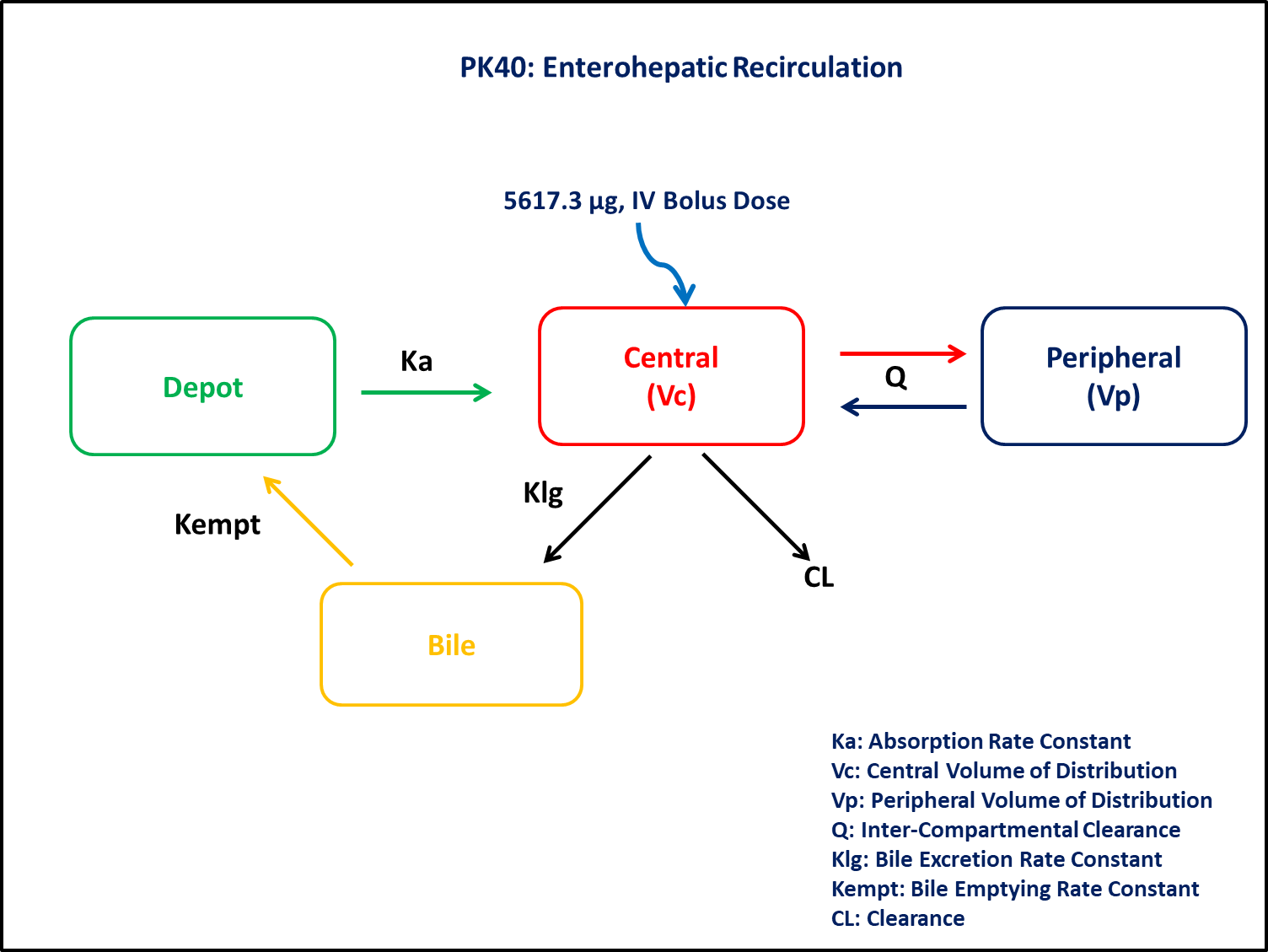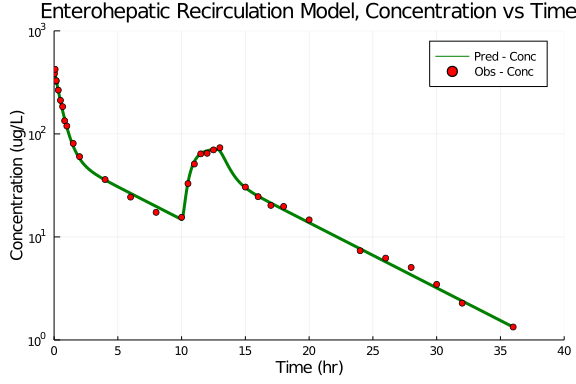# Exercise PK40 - Enterohepatic Recirculation

### Background

• Structural model - Enterohepatic Recirculation (EHS)

• Route of administration - IV Bolus dose

• Dosage Regimen - 5617.3 ug IV bolus dose was administered and drug plasma concentration was measured for 36 hrs

• Number of Subjects - 1 Subject### Libraries

call the "necessary" libraries to get started

using Pumas
using Plots
using CSV
using StatsPlots
using Random


### Model

It is multi-compartment Model with Enterohepatic Recirculation

pk_40            = @model  begin
@param begin
tvcl        ∈ RealDomain(lower=0)
tvvc        ∈ RealDomain(lower=0)
tvvp        ∈ RealDomain(lower=0)
tvQ         ∈ RealDomain(lower=0)
tvka        ∈ RealDomain(lower=0)
tvklg       ∈ RealDomain(lower=0)
tvτ         ∈ RealDomain(lower=0)
Ω           ∈ PDiagDomain(7)
σ²_prop     ∈ RealDomain(lower=0)
end

@random begin
η           ~ MvNormal(Ω)
end

@pre begin
Cl          = tvcl * exp(η)
Vc          = tvvc * exp(η)
Vp          = tvvp * exp(η)
Q           = tvQ * exp(η)
Ka          = tvka * exp(η)
Klg         = tvklg * exp(η)
τ           = tvτ * exp(η)
Kempt       = (t>10 && t<(10+τ))*(1/τ)

end

@dynamics begin
Central'    = Ka*Depot - (Cl/Vc)*Central + (Q/Vp)*Peripheral - (Q/Vc)*Central - Klg*Central
Peripheral' = (Q/Vc)*Central - (Q/Vp)*Peripheral
Bile'       = Klg*Central - Bile*Kempt
Depot'      = Bile*Kempt - Ka*Depot
end

@derived begin
cp          = @. Central/Vc
dv          ~ @. Normal(cp, sqrt(cp^2*σ²_prop))
end
end

PumasModel
Parameters: tvcl, tvvc, tvvp, tvQ, tvka, tvklg, tvτ, Ω, σ²_prop
Random effects: η
Covariates:
Dynamical variables: Central, Peripheral, Bile, Depot
Derived: cp, dv
Observed: cp, dv


### Parameters

Parameters are provided for the simulation as below. tv represents the typical value for parameters.

• tvcl - Clearance (L/hr)

• tvvc - Central Volume of Distribution (L)

• tvvp - Peripheral Volume of Distribution (L)

• tvQ - Intercompartmental Clearance (L/hr)

• tvka - Absorption rate Constant (hr⁻¹)

• tvklg - Bile Excretion rate constant (hr⁻¹)

• tvτ - Typical Value bile emptying Interval (hr)

• Kempt - Bile Emptying rate constant (hr⁻¹)

• Ω - Between Subject Variability

• σ - Residual error

param     =  (tvcl    = 0.842102,
tvvc    = 12.8201,
tvvp    = 29.0867,
tvQ     = 11.3699,
tvka    = 3.01245,
tvklg   = 0.609319,
tvτ     = 2.79697,
Ω       = Diagonal([0.0001,0.0001,0.0001,0.0001,0.0001,0.0001,0.0001]),
σ²_prop = 0.005)

(tvcl = 0.842102, tvvc = 12.8201, tvvp = 29.0867, tvQ = 11.3699, tvka = 3.0
1245, tvklg = 0.609319, tvτ = 2.79697, Ω = [0.0001 0.0 … 0.0 0.0; 0.0 0.000
1 … 0.0 0.0; … ; 0.0 0.0 … 0.0001 0.0; 0.0 0.0 … 0.0 0.0001], σ²_prop = 0.0
05)


### Dosage Regimen

A dose of 5617.3 ug IV bolus dose is administered at time 0 and drug plasma concentration was measured for 36 hrs

ev1  = DosageRegimen(5617.3, time=0, cmt=1)
sub1 = Subject(id=1, events=ev1)

Subject
ID: 1
Events: 1


### Simulation

Simulate the plasma concentration after IV administration

Random.seed!(123)
sim1 = simobs(pk_40, sub1, param, obstimes=0:000.001:36)
df1  = DataFrame(sim1)


### Dataframe & Plot

Use the dataframe for plotting

df1_dv = filter(x -> x.time in [0.03,0.083,0.15,0.17,0.33,0.5,0.67,0.83,1,1.5,2,4,6,8,10,10.5,11,11.5,12,12.5,13,15,16,17,18,20,24,26,28,30,32,36], df1)

@df df1 plot(:time, :cp,
yaxis=:log, label= "Pred - Conc", xlabel="Time (hr)", ylabel="Concentration (ug/L)",
color=[:green], linewidth=3, title = "Enterohepatic Recirculation Model, Concentration vs Time",
xticks = [0,5,10,15,20,25,30,35,40], yticks = [1,10,100,1000], xlims=(0,40), ylims=(1,1000))
@df df1_dv scatter!(:time, :dv, yaxis=:log, label="Obs - Conc", color=[:red])